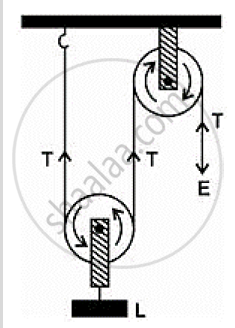Share

Books Shortlist

# The Mechanical Advantage of an Ideal Single Movable Pulley is 1 (B) 2 (C) Less than 2 (D) Less than 1? - ICSE Class 10 - Physics

#### Question

The mechanical advantage of an ideal single movable pulley is 1 (b) 2 (c) less than 2 (d) less than 1?

#### Solution

The mechanical advantage of an ideal single movable pulley is 2.
Derivation: Consider the diagram given below:Here the load L is balance by the tension in two segments of the string and the effort E balances the tension T at the free end, so
L = T + T = 2T and E = T
Assumption: Weight of the pulley is negligible.
We know that,
M.A ="𝐿𝑜𝑎𝑑 (𝐿)"/"𝐸𝑓𝑓𝑜𝑟𝑡 (𝐸)"= "2𝑇"/"𝑇"=2
Thus, a single movable pulley has a M.A. equal to 2.

Is there an error in this question or solution?

#### APPEARS IN

Solution The Mechanical Advantage of an Ideal Single Movable Pulley is 1 (B) 2 (C) Less than 2 (D) Less than 1? Concept: Concept of Pulley System.
S